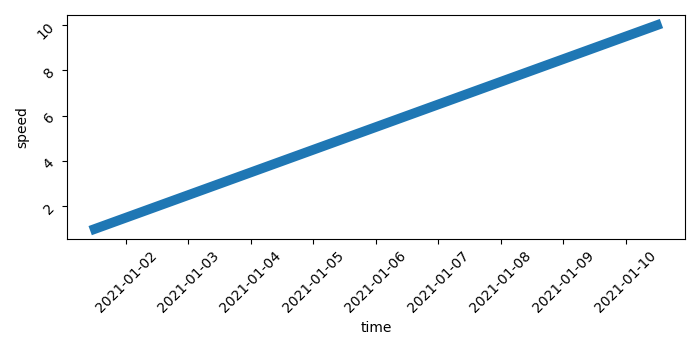# How to increase the line thickness of a Seaborn Line?

MatplotlibPythonData Visualization

To increase the line thickness of a Seaborn line, we can take the following steps

## Steps

• Set the figure size and adjust the padding between and around the subplots.

• Create a dataframe, df, of two-dimensional, size-mutable, potentially heterogeneous tabular data.

• Create a Seaborn line plot with linewidth value in the argument. Here we have set linewidth=7.

• Rotate the tick params, i.e., labels by 45 degrees.

• To display the figure, use Show() method.

## Example

import seaborn as sns
from matplotlib import pyplot as plt
import pandas as pd
import numpy as np

plt.rcParams["figure.figsize"] = [7.00, 3.50]
plt.rcParams["figure.autolayout"] = True

df = pd.DataFrame(
dict(
time=list(pd.date_range("2021-01-01 12:00:00", periods=10)),
speed=np.linspace(1, 10, 10)
)
)

ax = sns.lineplot(x="time", y="speed", data=df, lw=7)

ax.tick_params(rotation=45)

plt.show()

## Output

It will produce the following output −